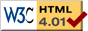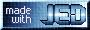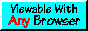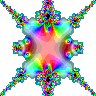# Complex Domain Coloring Gallery

The following figures were created using fztopng. The code that was fed into fztopng is shown to the left of the corresponding image.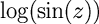define f_of_z (z) { return log(sin(z)); } define set_options (prefs) { prefs.xgrid = [-PI:2*PI:#384]; prefs.ygrid = [-1.5*PI:1.5*PI:#384]; prefs.iter = 11; }define f_of_z (z) { z + z^2/sin(z^4-1); } define set_options (prefs) { prefs.xgrid = [-1.5:1.5:#384]; prefs.ygrid = [-1.5:1.5:#384]; prefs.iter = 3; }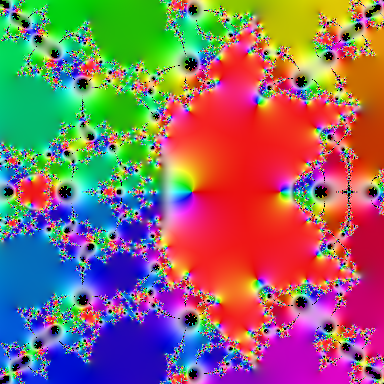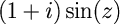define f_of_z (z) { (1+1i)*sin(z); } define set_options (prefs) { prefs.xgrid = [-1.5:1.5:#384]; prefs.ygrid = [-1.5:1.5:#384]; prefs.iter = 7; prefs.method = RGB_METHOD_GRADIENT; }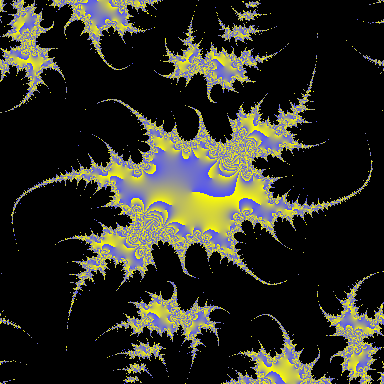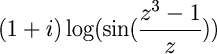define f_of_z (z) { (1+1i)*log(sin(z^3-1)/z); } define set_options (prefs) { prefs.xgrid = [-1.5:1.5:#384]*1.5; prefs.ygrid = [-1.5:1.5:#384]*1.5; prefs.iter = 0; prefs.hue=[-90,270]; }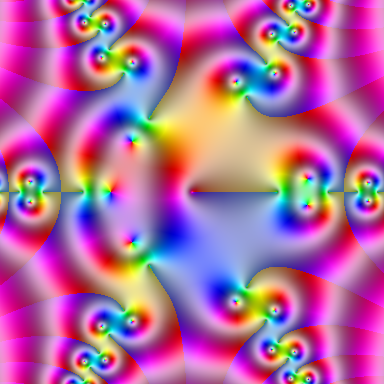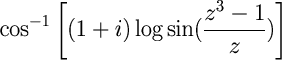define f_of_z (z) { acos ((1+1i)*log(sin(z^3-1)/z)); } define set_options (prefs) { prefs.xgrid = [-1.5:1.5:#384]*2; prefs.ygrid = [-1.5:1.5:#384]*2; prefs.iter = 1; prefs.hue=[-180,180]; }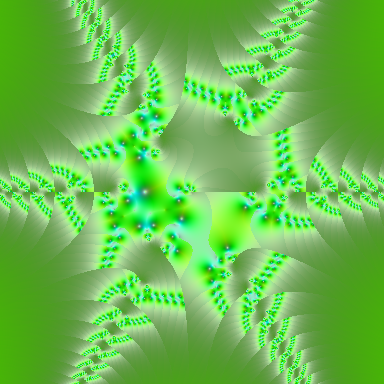This page was last updated Nov 26, 2017 by John E. Davis. To comment on it or the material presented here, send email to jed at jedsoft org.